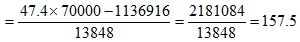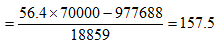Answer question nos. 1-3 based on the following data:
The retail prices of flowers, consumer expenditure on flowers and sales of flowers for the calendar year 2009, in Phoolgaon, a small town with a population of 70000, is summarized in table 2.
Table 2: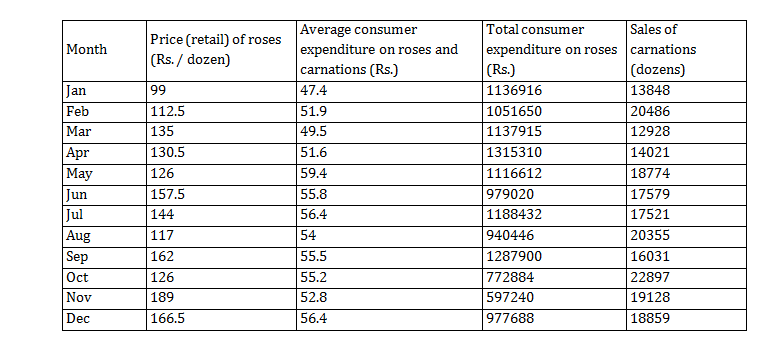Question 1.  Compared to January, the total expenditure on carnations in March
A. increased by 6.27%
B. decreased by 6.64%
C. increased by 6.69%
D. decreased by 7.11%
E. did not change

Question 2. Compared to January, the sales of roses in July
A. decreased by 39.15%
B. decreased by 28.13%
C. increased by 4.53%
D. increased by 4.33%
E. did not change

Question 3. Compared to January, the price of carnations in December
A. increased by 26.57%
B. increased by 28.12%
C. increased by 36.19%
D. increased by 38.16%
E. did not change

Total expenditure on carnations in January

= 47.4 × 70000 – 1136916 = 2181084

And total expenditure on carnations in March

= 49.5 × 70000 – 1137915 = 2327085

So percentage increase =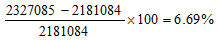Sales of roses in January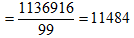Sales of roses in July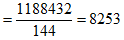∴   Percentage decrease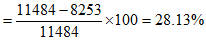Hence, option (B) is the correct answer.